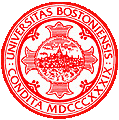# HW2: Programming Assignment

CS 585 HW 2
Guangxing Ren
Yueying Li
Xi You
26/09/2018

## Problem Definition

This Problem require our team to design and implement algorithms that can analyze and recognize random shape The shapes are categorized into three types: square, circle and equilateral triangle.
We have five specific problems:

1.For each shape image, determine its background color and label each and every shape blobs according to their color.
2.For each shape blob, implement a border following algorithm to find its outermost contour (i.e. the border pixels) and compare it with OpenCV's "findContours" function.
3.For each shape blob, classify its border pixels (in other words, segment its outermost contour) into three types: borders against the background, borders against another shape blob and borders that are a border of the whole image.
4.(Optional) You may also segment the border according to their convexity, i.e. find out all convex segments and concave segments. This may help you analyze its shape type.
5.For each shape blob, come up with an algorithm that can recognize its shape type (square, circle, or triangle).

## Method and Implementation

Problem 1 Method:
We traverse the whole image twice. For the first time, we use a colorMap to record every pixel’s color. After that, we merge some color according to the threshold. And for the second time we compare each color in the colorMap with the current pixel and mark each pixel by the color they match. Finally, the output of the function is an image marked by object’s label and a vector contained the points of each object.
Problem 2 Method:
For the second problem, we use Moore-Neighbor Tracing Algorithm to solve the problem. The link attaches on the bottom of the page. We only introduce briefly at this part.
1. Find a start pixel named point c
2. Find the N4(N, W, S, E) neighbor of c and mark the west pixel of c as b
3. Among N8(NW, N, NE, W, E, SW, S, SE) neighbor of c, starting with b, search clockwise to look for the first boundary pixel.
4. Let b becomes the new c and the previous b becomes the new b
5. Repeat 3&4 until c becomes the start cProblem 3 Method:
Find the average value(central point) of the points on the coincident edge(of two objects for example, object 1 and object 2). If the point of average value on the object 1(the value of central point equals object 1), the object 1 blocks the object 2. If point on the object 2, the object 2 blocks the object 1. This object can be the object on the image or the boundary.
problem 4 method:
In Problem 3 we find out the average points on the coincident edge, If the point of average value on the object 1, this segement is a convex part of object 1, on the other hand, this segement is a convex part of object 1 concave part of object 1.
Problem 5 Method:
For this problem we find leftmost point, rightmost point, top point and bottom point. And then we connect each point to calculate the degree of their angle. If one of the angle is 90 degree we mark it as square and if one of the angle is 60 degree we mark it as equilateral triangle and if it's none of them we mark it as circle.

## Experiments

Experiments Environment: window 10/visual studio 2017
Size of the image: 128p*128p

Our method can be applied to the png image provided in each job, and some of them are applicable to the provided jepg image.

## Results

trial 1 1027.png
orginal imagep1as you can see, we successfully label 5 color
p2
opencv findcontourdiy findcontouras you can see,our accuracy is 0.28, although the number is not high but we find all contours and visually matching.
p3as you can see, we recongize which object is being block and which is a whole imageobject segement that against each other mark RED
p4we correctly recognize three of four object shape

## Discussion

Discuss your method and results:

• What are the strengths and weaknesses of your method? The strength of the method I use is entirely base on the theory so it's easy to understand. But it's time-wasting, you have to traverse the whole image.
• If I choose the other methods I mentioned above for grayscale, the brightness of this image will change a little bit but it can be observed.
• In future work, I want to figure out more ways to deal with image and turn it into other style

## Conclusions

The color and position of the image can be transformed by changing the RGB value and the position of the pixel.The image in real life is hard to process as it is not perfect.

## Credits and Bibliography

The solution of problem one got the suggestion from Xueying Han
Moore-Neighbor Tracing https://www.cnblogs.com/biglucky/p/4136946.html
Template of this homework: http://www.cs.bu.edu/faculty/betke/cs585/restricted/hw-instructions/cs585-homework-template.html

All the problems came across during the possess discussed with Yueying Li and Xi You